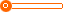# 向大大厦门华博基尼 蕾丝款 无钢圈内衣 文胸套装 超聚拢 哺乳内衣 清仓甩卖

1 套

2000 套

(发货期限：自买家付款之日起 天内发货)

• VIP指数:[第1年]
• 认证信息:
• 所在地区：福建
•• 详细说明
• 规格参数
• 联系方式
r

r r

r  正品向大大内衣，微商转行，多款内衣低价清仓，需要可以加V信：13666009389r

r

r 也可直接进入TB店铺咨询购买：r

r

r 复制链接进入r

rr

r

r r

r

r   r

r

r 我们的棉统一为记忆棉，没有海绵，放心，保证没有海绵！！！ r

r

r
r

r

r 我们的优势：具有极强的聚拢效果，防下垂光滑无痕，零甲醛，健康舒适，收副乳，收外扩，调整胸型，贴身不跑杯，透气柔软排湿性强，性价比超 r

r

r   r

r

r 好处：改善女人的胸型，健康舒适，无束缚感，独特效果帮助发育不良，怀孕和哺乳期囤积的脂肪恢复，使肌纤维强韧上挺，增强肌肤弹性促进血液循环，使乳房变光滑力挺柔润，一片式立体剪裁，侧面及背部均无压力。 r

r

r
r

r

r
r

r

r
r

r

r 聚拢胸器产品介 r

r

r 我们穿的不是内衣，我们穿的是惊艳！ r

r

r 1.产品类别：文胸套装(文胸+三角内裤） r

r

r 2.面料类型：棉纶+氨纶 r

r

r 3.功能效果:无痕聚拢按摩上拖侧收副乳舒适透气 r

r

r 4.排扣类型：后四排三组搭扣 r

r

r 5.肩带类型：可拆卸肩带 r

r

r 6.模杯类型：上薄下厚，无钢圈，加强上拖功效 r

r

r 7.风格类型：性感妩媚 r

r

r 8.设计特色：光面一片式底心位 r

r

r 我们只卖正品，请认准厦门华博基尼有限公司本产品100%华博基尼NB正品.支持验货若发现是冒牌伪劣产品.承诺假一赔十！欢迎大家验货~ r

r

r
r

r

r 扫一扫加我了解更多~ r

r

r
r
r

r

r r

r

r
r
r
r
r
r

r

### r 如果你有空余的时间，需要兼职赚点外快的话，可联系我做微信代理！ r

r

r 为了第一时间回复您的消息，请加微信：13666009389 详谈  r

r"};

查看更多同类品牌产品
上一组 下一组

## 您可以通过以下类目找到类似信息：

免责声明：以上所展示的信息由会员自行提供，内容的真实性、准确性和合法性由发布会员负责。生活头条网对此不承担任何责任。

友情提醒：为规避购买风险，建议您在购买相关产品前务必确认供应商资质及产品质量！